# Simplification Techniques and Tricks - PDF

The topic Simplification is asked in every exam. In bank exams, Simplification covers almost 30-40% of the Quantitative Aptitude section. Simplification also covers the percentage chapter. Simplification topic is asked to check the ability of students to find their ability to deal with the numbers. So, it’s important to have knowledge about simplification. Let’s try to understand Simplification-

Simplification means to play with the questions by doing complex calculations. By using following of the tricks and tactics you can score good by saving your valuable time:-
• Learn BODMAS.
• Use the concept of digital sum.
• Memorize tables up to 30.
• Memorize cubes and squares of numbers up to 35.
• Learn tricks to find squares and cubes of numbers greater than 35.
• Learn tricks to find cube roots and square roots of large number.
• Learn the concept of percentages (conversion of fractions to percentage & percentage to fractions)
• Memorize the reciprocals.

Learn Fast Maths Tricks here

Rules of Simplification
V → Vinculum
B → Remove Brackets - in the order ( ) , { }, [ ]
O → Of
D → Division
M → Multiplication
S → Subtraction

Let’s discuss an example based on BODMAS -

### Example: 152 × 23 + (228 ÷ 19)2 =?

Sol:
⇒ 15✕(12)2 [brackets are solved first and table of 19 and 15 must be on tips]
⇒ 120+144 [must know the squares]
⇒ 264

### Important Parts of Simplification

• Digital Sum
• Number System
• Reciprocals
• HCF & LCM
• Percentages
• Square & Cube
• Approximation
• Fractions & Decimals
• Surds & Indices

## Digital Sum

Digital sum is the sum obtained after adding all the digits of any given number successively.
• Example: 568 = 5+6+8 = 19, 1 + 9 = 10.
Note: if any number multiplied by 9, then the digital sum is always 9.
• Example: 6 ✕ 9 = 54, 5+4 = 9
Trick: In order to save time if we find digit 9 or multiples of 9, then 9 or its multiple can be neglected.
• Example: 293 = 2 + 9 + 3 = 2 + 3 = 5 [ ‘9’ is omitted ]
‘9’ is omitted to reduce the calculation.
If we don’t omit ‘9’, then also the digital sum remains same.
Example: 293 = 2 + 9 + 3 = 14, 1 + 4 = 5 [answer remains same]
Let’s discuss one more example-

### Example: 326 ✕ 890 =?

a. 291140
b. 290100
c. 290140
d. 293990
Sol: We can find out the answer by option method without doing multiplication. This is only possible with the help of Digital sum.

Now, Digital sum, 326  890 = (3 + 2 +6)  ( 8 +9 + 0 )
⇒ 11 ✕ 17
⇒ (1+1) ✕ (1+7)
⇒ 2 ✕ 8 = 16
⇒ digital sum (16) = 7
Now find out the digital sum of the given options-
1. DS (291140) = 8
2. DS (290100) = 3
3. DS (290140) = 7
4. DS (293990) = 5
Option C has the same digital sum as ‘7’ as we have already found out. Thus the correct option is C.

## Number System

• Classification
• Divisibility Test
• Division& Remainder Rules
• Sum Rules

#### Classification

Types Description
Natural Numbers:
all counting numbers ( 1,2,3,4,5....∞)
Whole Numbers:
natural number + zero( 0,1,2,3,4,5...∞)
Integers:
All whole numbers including Negative number + Positive number(∞......-4,-3,-2,-1,0,1,2,3,4,5....∞)
Even & Odd Numbers :
All whole number divisible by 2 is Even (0,2,4,6,8,10,12.....∞) and which does not divide by 2 are Odd (1,3,5,7,9,11,13,15,17,19....∞)
Prime Numbers:
It can be positive or negative except 1, if the number is not divisible by any number except the number itself.(2,3,5,7,11,13,17,19,23,29,31,37,41,43,47,53,59,61....∞)
Composite Numbers:
Natural numbers which are not prime
Co-Prime:
Two natural number a and b are said to be co-prime if their HCF is 1.

#### Divisibility

Numbers IF A Number Examples
Divisible by 2 End with 0,2,4,6,8 are divisible by 2 254,326,3546,4718 all are divisible by 2
Divisible by 3 Sum of its digits  is divisible by 3 375,4251,78123 all are divisible by 3.  [549=5+4+9][5+4+9=18]18 is divisible by 3  hence 549 is divisible by 3.
Divisible by 4 Last two digit divisible by 4 5648 here last 2 digits are 48 which is divisible by 4 hence 5648 is also divisible by 4.
Divisible by 5 Ends with 0 or 5 225 or 330 here last digit digit is 0 or 5 that mean both the numbers are divisible by 5.
Divisible by 6 Divides by Both 2 & 3 4536 here last digit is 6 so it divisible by 2 & sum of its digit (like 4+5+3+6=18) is 18 which is divisible by 3.Hence 4536 is divisible by 6.
Divisible by 8 Last 3 digit divide by 8 746848 here last 3 digit 848 is divisible by 8 hence 746848 is also divisible by 8.
Divisible by 10 End with 0 220,450,1450,8450 all numbers has a last digit zero it means all are divisible by 10.
Divisible by 11 [Sum of its digit in  odd places-Sum of its digits in even places]= 0 or multiple of 11
Consider the number 39798847
(Sum of its digits at odd places)-(Sum of its digits at even places)
(7+8+9+9)-(4+8+7+3)
(23-12)
23-12=11,
which is divisible by 11. So 39798847 is divisible by 11.

#### Division & Remainder Rules

Suppose we divide 45 by 6hence ,represent it as:
dividend = ( divisorquotient ) + remainder
or
divisior= [(dividend)-(remainder] / quotient
could be write it as
x = kq + r where (x = dividend,k = divisor,q = quotient,r = remainder)

Example:
On dividing a certain number by 342, we get 47 as remainder. If the same number is divided by 18, what will be the remainder ?
Number = 342k + 47
( 18 19k ) + ( 18 2 ) + 11
18 ( 19k + 2 ) +11.
Remainder = 11

#### Sum Rules

(1+2+3+.........+n) = 1/n(n+1)
(12+22+32+.........+n2) = 1/n (n+1) (2n+1)
(13+23+33+.........+n3) = 1/4 n2 (n+1)2
Arithmetic Progression (A.P.)
a, a + d, a + 2d, a + 3d, ....are said to be in A.P. in which first term = a and common difference = d.
Let the nth term be tn and last term = l, then
a) nth term = a + ( n - 1 ) d
b) Sum of n terms = n/2 [2a + (n-1)d]
c) Sum of n terms = n/2 (a+l) where l is the last term

## Reciprocals

Up to 5, the reciprocals are easy to remember. But after 5 are explained as below-

• 1/7 = 0.142857
• 2/7 = 0.285714
• 3/7 = 0.42857
• 5/7 = 0.714285
• 6/7 = 0.857142
• 1/8 = 0.125
• 2/8 = ¼ = 0.25
• 3/8 = 3 × 1/8 = 0.375
• 4/8 = ½ = 0.5
• 5/8 = 4/8 + 1/8 = 0.5 + 0.125 = 0.625
• 6/8 = ¾ = 0.75
• 7/8 = 6/8 + 1/8 = 0.75 + 1.25 = 0.875
• 1/9 = 0.1111…
• 2/9 = 0.2222…
• 3/9 = 0.3333…
• 1/11 = 0.090909…
• 2/11 = 0.181818…
• 3/11 = 0.272727…
• 10/11 = 0.909090…
• 1/12 = ½ × 1/6 = ½ × 0.166666 = 0.083333…
• 1/13 = 0.076923
• 1/14 = 0.071428
• 1/15 = 1/3 × 1/5 = 0.33333 × 0.2
• 1/15 = 0.0666…
• 1/16 = ½ × 1/8 = ½ × 0.125 = 0.0625
• 1/17 = 0.058823
• 1/18 = ½ × 1/9 = ½ × 0.111111 = 0.0555…
• 1/19 = 0.052631
• 1/20 = 0.05

## H.C.F. & L.C.M.

• Factorization & Division Method
• HCF & LCM of Fractions & Decimal Fractions

#### Methods

On Basis
H.C.F. or G.C.M
L.C.M.
Factorization Method
Write  each number as the product of the prime factors. The product of least powers of common prime factors gives H.C.F.
Example:
Find the H.C.F. of 108, 288 and 360.
108 = 22✘33, 288 = 25✘32 and 360 = 23✘5✘32
H.C.F. = 22✘32=36
Write each numbers into a product
of prime factors. Then, L.C.M is
the product of highest powers of
all the factors.
Examples:
Find the L.C.M. of 72, 108 and 2100.
72=23✘32,108=33✘22,
2100=22✘52✘3✘7.
L.C.M.=23✘33✘52✘7=37800
Division Method
Let we have two numbers .Pick the smaller one and divide it by the larger one. After that divide the divisor with the remainder. This process of dividing the preceding number by the remainder will repeated until we got the zero  as remainder.The last divisor is the required H.C.F.
Example: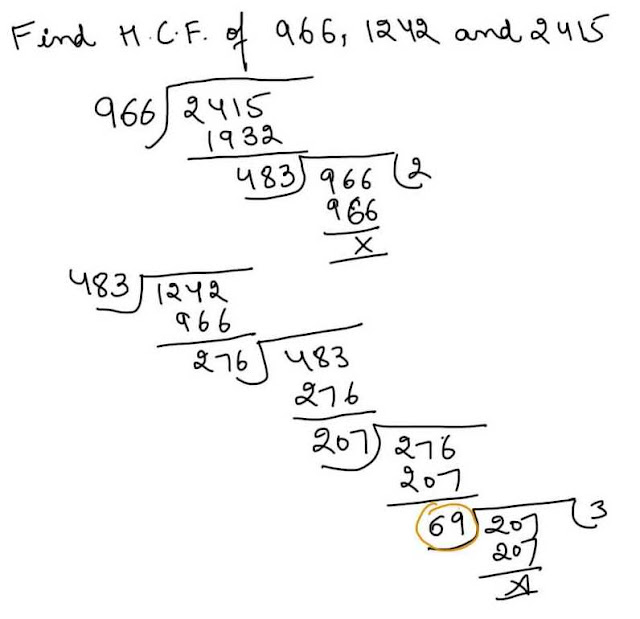H.C.F. of given numbers = 69
Let we have set of numbers.
First of all find the number
which divide at least two of
the number in a given set of
number.remainder and
not divisible numbers
will carry forward as it is.
Repeat the process till
at least  two number is
not divisible by any number
except 1.The product of
the divisor and the
undivided numbers is the required
L.C.M.
Example:
Find the L.C.M. of 12,36,48,72
H.C.F. & L.C.M. of Fractions
H.C.F. =  H.C.F. of Numerator / L.C.M. of Denominators L.C.M. = L.C.M. of Numerator / H.C.F. of Denominators
Product of H.C.F. & L.C.M.
H.C.F * L.C.M. = product of two numbers
Decimal numbers H.C.F. of Decimal numbers
Step 1. Find the HCF of the given numbers without decimal.
Step 2.Put the decimal point ( in the HCF of Step 1) from right to left according to the MAXIMUM deciaml places among the given numbers.
L.C.M. of Decimal numbers
Step 1. Find the LCM of the given numbers without decimal.
Step 2.Put the decimal point ( in the LCM of Step 1) from right to left according to the MINIMUM deciaml places among the given numbers.

## Percentages

Percent implies “for every hundred”. % is read as percentage and y % is read as y per cent.
• Calculation of q % of z
• (q/100) ✕ z = (q ✕ z)/100
• q % of z = z % of q

### Percentage – Ratio Equivalence:

#### Example:12.5% of 800

Sol: 1/8 ✕ 800 [12.5% = 1/8, Learn the ratio- equivalence table]
⇒100

## Square & Cube

• Square & Cube
• Square Root & Cube Root
• Factorization Method
 Perfect Square Non-Perfect Square last digit is 1, 4, 9, 6, 5 last digit is 2, 3, 7, 8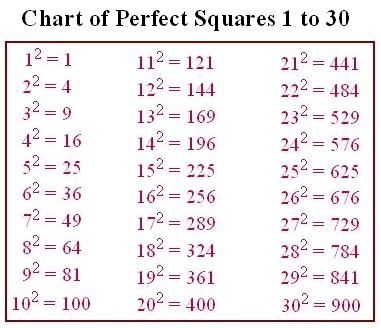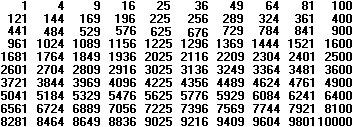Square Root & Cube Root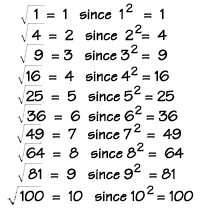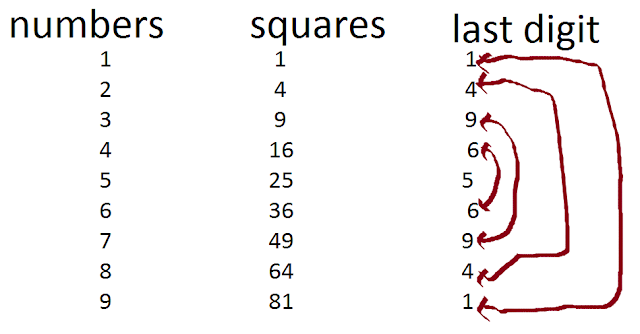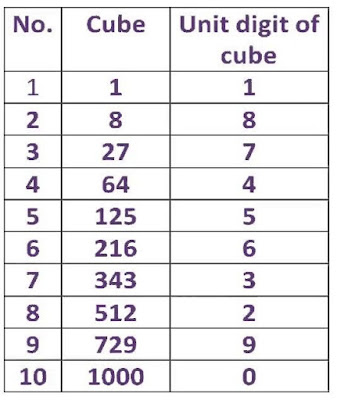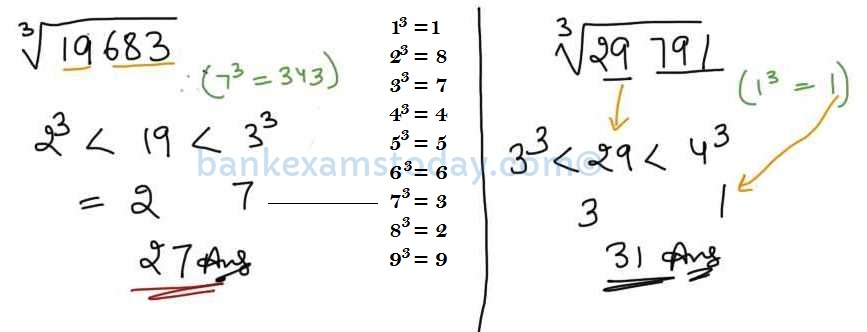## Approximation

As the name suggests, if the given values are in points, then approximate the values to the nearest comfortable value so that there is not much effect on the final results.
Example: 150.4  5.876
Sol: 1500 ✕ 6 =9000

## Fractions & Decimals

On Basis Explanation
Decimal Fractions
A number with a denominator of power of 10 is a decimal fractions.
1/10= 1 tenth; 1/100= 0.1;38/100=0.38
Vulgar Fractions
Conversion of 0.64(decimal number) into a Vulgar Fraction.First of all write the numeric digit 1 in the denominator of a number (like here 0.64) and add as many numeric zeros as the digit in the number after decimal point.After that removes the decimal point from the given number.At last step just reduce the fraction to its lowest terms. So, 0.64 = 64/100=16/25;25.025 = 25025/1000 = 1001/4
To perform the addition and subtraction of a decimal fraction could be done through placing them right under each other that the decimal points lie in one column.
3.424+3.28+.4036+6.2+.8+4
3. 424
3. 28
. 4036
6. 2
. 8
+4______
18. 1076

Multiplication of a Decimal Fraction
To find the multiplication of decimal fraction , first of all you need to remove the decimal point from the given numbers and then perform the multiplication after that assign the decimal point as many places after the number as the sum of the number of the decimal places in the given number.
Step 1. 0.06*0.3*0.40
Step 2. 6*3*40=720
Step 3. 0.00720
Multiplication of a decimal fraction by power of 10
A multiplication of a decimal fraction by a power of 10 can be perform through shifting the decimal point towards right as many places as is the power of 10.
like 45.6288*100=45628.8, 0.00452*100=0.452
DivisionComparison of Fractions To compare the set of fractions numbers,first of all you need to convert each fraction number or value into a equal decimal value and then it will be became easy for you to assign them ( the numbers or value) in a particular way( ascending or descending order).
3/5,4/7,8/9 and 9/11 Arranging in Ascending Order
3/5= 0.6, 4/7 = 0.571, 8/9 = 0.88, 9/11 = 0.818.
Now, 0.88 > 0.818 > 0.6 > 0.571
8/9>9/11>3/5>4/7
Recurring Decimal Recurring Decimal
A decimal number in which after a decimal point a number or set of number are repeated again and again are called recurring decimal numbers.It can be written in shorten form by placing a bar or line above the numbers which has repeated.Pure Recurring Decimal
A decimal number in which all digits are repeated after a decimal point.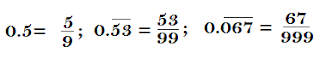Mixed Recurring Decimal
A decimal number in which certain digits are repeated only.### Surds & Indices

• Some Rules of Indices
• Some Rules of SurdsNow let’s discuss some questions based on the above tricks:

### 1. (9.979)3 – (23.99)2 + (1.99)5 =?

Sol: Using the concept of approximation,
⇒ 103 – 242 + 25 = 1000 – 576 – 32
⇒ 456

### 2. 25% of 2500 + 16.66%% of 144 =?

Sol: 25/100 ✕ 2500 + 1/6 ✕ 144 [16.66% =1/6]
⇒ 252 + 24 = 625 + 24
⇒ 649

### 3. ∛7.938 x∛999.9998– 4.9256 =?

Sol: Using the concept of approximation,⇒ 2✕10-5 [must know the cubes, then you can also find out cube roots & square--roots easily]
⇒ 15

### 4. (125)1.3 × (25)1.8 ÷ (5)0.5 = (5)?

Sol: (5)3×1.3 ✕ (5)2×1.8(5)0.5 = (5)?
⇒ (5)3.9 ✕ (5)3.6 ÷ (5)0.5 = (5)?
⇒ (5)3.9+3.6-0.5 = (5)?
⇒ ? = 7.5 – 0.5 = 7

### 5. 13 × 2 (65 – 15) + 168 ÷ 2 ÷ 2- (11)2 =?

Sol: Using the concept of BODMAS,
⇒ 13 ✕ 2 ✕ 50 + 168/2 2 – 121 =?
⇒ 1300 + 84/2 – 121 =?
⇒ 1300 + 42 – 121
⇒ 1221

Use the above tricks with accuracy in order to increase your speed. So, practice as much as you can. Set a timer and make sure yourself that you have to attempt 10-12 questions within 4-5 minutes while practising and if you get failed in doing this, then practice again and again.

### Simplification Shortcuts PDF

Number of pages - 53
File format - PDF
Price - Free
File-size - 1.7 mb